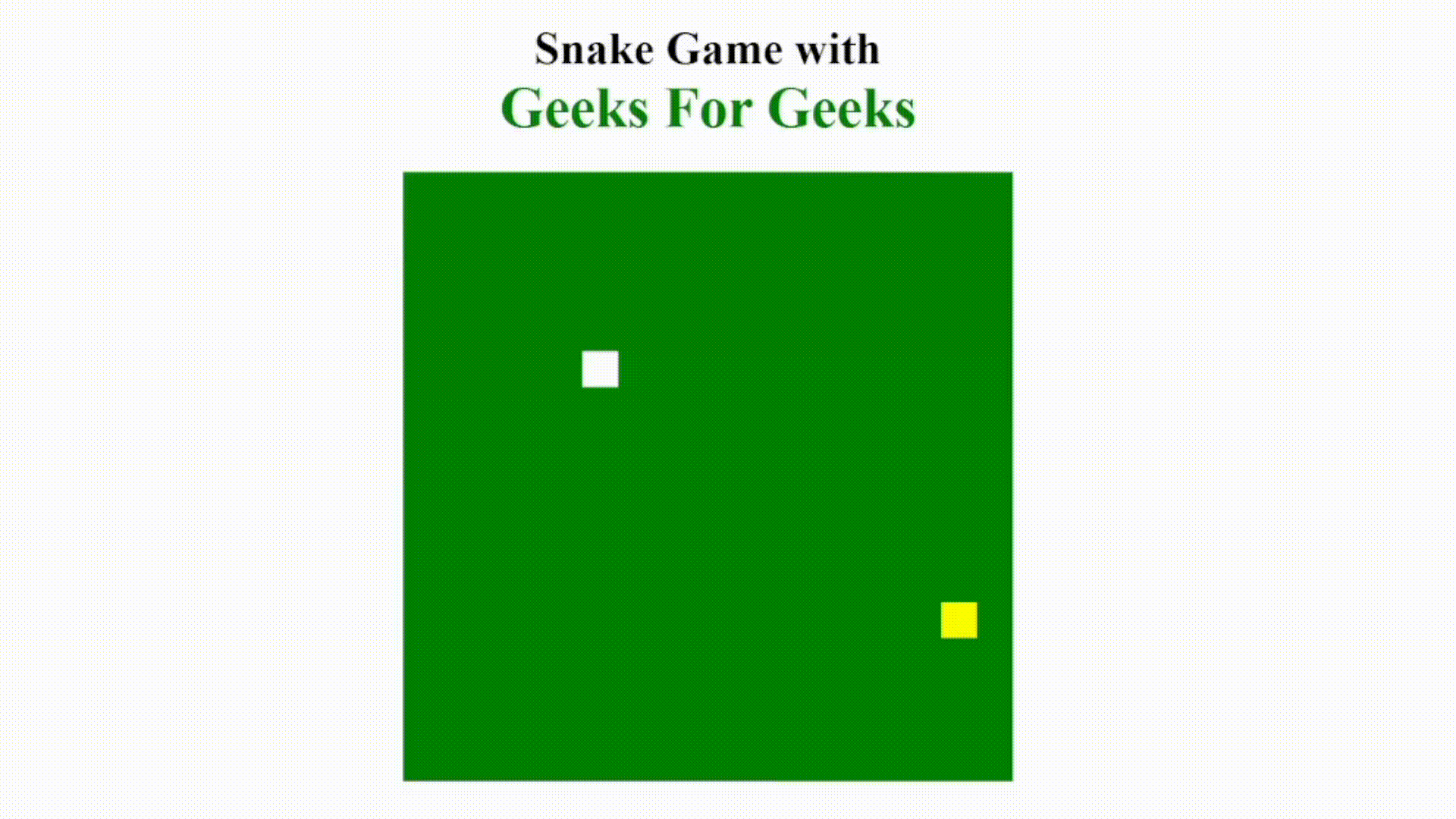GeeksforGeeks App
Open AppBrowser
Continue

# Create a snake game using HTML, CSS and JavaScript

Snake Game is a single-player game where the snake gets bigger by eating the food and tries to save itself from the boundary of the rectangle and if the snake eats their own body the game will be over.

We are going to build a Snake Game using HTML, CSS, and JavaScript.

Prerequisite:

Game Rules:

• If the snake goes out of the boundary or eats its own body the game will be over.

Approach:  We will create three files (an HTML file, a CSS file, and JavaScript File) we will give styling in the CSS file and functionality in the JavaScript part. Movement and food will be created in a JavaScript file. We will be implementing the functionality mentioned below:

• Select the board id from the HTML and add functionality to that board using JavaScript like board size, snake color, food color, Snake size, food size snake position.
• Create the background of a game using the JavaScript fillstyle() method.
• Place food on the board using Math.random().
• Select the speed of the snake using setInterval().

Implementation: Write the code into working with the approach as discussed above:

index.html

## HTML

 ```<``html``>` `<``head``>``    ``<``meta` `charset``=``"UTF-8"``>``    ``<``meta` `name``=``"viewport"``,``          ``content``=``"width=device-width, initial-scale=1.0"``>``    ``<``title``>Snake Game with GFG``    ``<``link` `rel``=``"stylesheet"` `href``=``"style.css"``>``    ``<``script` `src``=``"script.js"``>``` `<``body``>``    ``<``h1``>Snake Game with``          ``<``div` `class``=``"geeks"``>Geeks For Geeks``    ````    ``<``canvas` `id``=``"board"``>``` ``

style.css

## CSS

 `/* Write CSS Here */``body {``    ``text-align``: ``center``;``}``.geeks {``    ``font-size``: ``40px``;``    ``font-weight``: ``bold``;``    ``color``: ``green``;``}`

script.js:

## Javascript

 `var` `blockSize = 25;``var` `total_row = 17; ``//total row number``var` `total_col = 17; ``//total column number``var` `board;``var` `context;` `var` `snakeX = blockSize * 5;``var` `snakeY = blockSize * 5;` `// Set the total number of rows and columns``var` `speedX = 0;  ``//speed of snake in x coordinate.``var` `speedY = 0;  ``//speed of snake in Y coordinate.` `var` `snakeBody = [];` `var` `foodX;``var` `foodY;` `var` `gameOver = ``false``;` `window.onload = ``function` `() {``    ``// Set board height and width``    ``board = document.getElementById(``"board"``);``    ``board.height = total_row * blockSize;``    ``board.width = total_col * blockSize;``    ``context = board.getContext(``"2d"``);` `    ``placeFood();``    ``document.addEventListener(``"keyup"``, changeDirection);  ``//for movements``    ``// Set snake speed``    ``setInterval(update, 1000 / 10);``}` `function` `update() {``    ``if` `(gameOver) {``        ``return``;``    ``}` `    ``// Background of a Game``    ``context.fillStyle = ``"green"``;``    ``context.fillRect(0, 0, board.width, board.height);` `    ``// Set food color and position``    ``context.fillStyle = ``"yellow"``;``    ``context.fillRect(foodX, foodY, blockSize, blockSize);` `    ``if` `(snakeX == foodX && snakeY == foodY) {``        ``snakeBody.push([foodX, foodY]);``        ``placeFood();``    ``}` `    ``// body of snake will grow``    ``for` `(let i = snakeBody.length - 1; i > 0; i--) {``        ``// it will store previous part of snake to the current part``        ``snakeBody[i] = snakeBody[i - 1];``    ``}``    ``if` `(snakeBody.length) {``        ``snakeBody = [snakeX, snakeY];``    ``}` `    ``context.fillStyle = ``"white"``;``    ``snakeX += speedX * blockSize; ``//updating Snake position in X coordinate.``    ``snakeY += speedY * blockSize;  ``//updating Snake position in Y coordinate.``    ``context.fillRect(snakeX, snakeY, blockSize, blockSize);``    ``for` `(let i = 0; i < snakeBody.length; i++) {``        ``context.fillRect(snakeBody[i], snakeBody[i], blockSize, blockSize);``    ``}` `    ``if` `(snakeX < 0``        ``|| snakeX > total_col * blockSize``        ``|| snakeY < 0``        ``|| snakeY > total_row * blockSize) {``        ` `        ``// Out of bound condition``        ``gameOver = ``true``;``        ``alert(``"Game Over"``);``    ``}` `    ``for` `(let i = 0; i < snakeBody.length; i++) {``        ``if` `(snakeX == snakeBody[i] && snakeY == snakeBody[i]) {``            ` `            ``// Snake eats own body``            ``gameOver = ``true``;``            ``alert(``"Game Over"``);``        ``}``    ``}``}` `// Movement of the Snake - We are using addEventListener``function` `changeDirection(e) {``    ``if` `(e.code == ``"ArrowUp"` `&& speedY != 1) {``        ``// If up arrow key pressed with this condition...``        ``// snake will not move in the opposite direction``        ``speedX = 0;``        ``speedY = -1;``    ``}``    ``else` `if` `(e.code == ``"ArrowDown"` `&& speedY != -1) {``        ``//If down arrow key pressed``        ``speedX = 0;``        ``speedY = 1;``    ``}``    ``else` `if` `(e.code == ``"ArrowLeft"` `&& speedX != 1) {``        ``//If left arrow key pressed``        ``speedX = -1;``        ``speedY = 0;``    ``}``    ``else` `if` `(e.code == ``"ArrowRight"` `&& speedX != -1) {``        ``//If Right arrow key pressed``        ``speedX = 1;``        ``speedY = 0;``    ``}``}` `// Randomly place food``function` `placeFood() {` `    ``// in x coordinates.``    ``foodX = Math.floor(Math.random() * total_col) * blockSize;``    ` `    ``//in y coordinates.``    ``foodY = Math.floor(Math.random() * total_row) * blockSize;``}`

Output:Snake Game with GFG

My Personal Notes arrow_drop_up
Related Tutorials